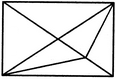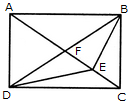# Non Verbal Reasoning - Analytical Reasoning - Discussion

Discussion Forum : Analytical Reasoning - Section 1 (Q.No. 31)
31.

Find the number of triangles in the given figure.11
13
15
17
Explanation:

The figure may be labelled as shown.The simplest triangles are AFB, FEB, EBC, DEC, DFE and AFD i.e. 6 in number.

The triangles composed of two components each are AEB, FBC, DFC, ADE, DBE and ABD i.e. 6 in number.

The triangles composed of three components each are ADC and ABC i.e. 2 in number.

There is only one triangle i.e. DBC which is composed of four components.

Thus, there are 6 + 6 + 2 + 1 = 15 triangles in the figure.

Discussion:
2 comments Page 1 of 1.

Santosh Yadav said:   7 years ago
Nice method. Thanks for giving this question.

Abdul said:   3 years ago
Thanks for the information.